Playfair Cipher with Examples

• Difficulty Level : Easy
• Last Updated : 24 Dec, 2021

The Playfair cipher was the first practical digraph substitution cipher. The scheme was invented in 1854 by Charles Wheatstone but was named after Lord Playfair who promoted the use of the cipher. In playfair cipher unlike traditional cipher we encrypt a pair of alphabets(digraphs) instead of a single alphabet.
It was used for tactical purposes by British forces in the Second Boer War and in World War I and for the same purpose by the Australians during World War II. This was because Playfair is reasonably fast to use and requires no special equipment.

Encryption Technique

For the encryption process let us consider the following example:The Playfair Cipher Encryption Algorithm:
The Algorithm consists of 2 steps:

1. Generate the key Square(5×5):
• The key square is a 5×5 grid of alphabets that acts as the key for encrypting the plaintext. Each of the 25 alphabets must be unique and one letter of the alphabet (usually J) is omitted from the table (as the table can hold only 25 alphabets). If the plaintext contains J, then it is replaced by I.

• The initial alphabets in the key square are the unique alphabets of the key in the order in which they appear followed by the remaining letters of the alphabet in order.

2. Algorithm to encrypt the plain text: The plaintext is split into pairs of two letters (digraphs). If there is an odd number of letters, a Z is added to the last letter.
For example:

PlainText: "instruments"
After Split: 'in' 'st' 'ru' 'me' 'nt' 'sz'

1. Pair cannot be made with same letter. Break the letter in single and add a bogus letter to the previous letter.

Plain Text: “hello”

After Split: ‘he’ ‘lx’ ‘lo’

Here ‘x’ is the bogus letter.

2. If the letter is standing alone in the process of pairing, then add an extra bogus letter with the alone letter

Plain Text: “helloe”

AfterSplit: ‘he’ ‘lx’ ‘lo’ ‘ez’

Here ‘z’  is the bogus letter.

Rules for Encryption:

• If both the letters are in the same column: Take the letter below each one (going back to the top if at the bottom).
For example:

Diagraph: "me"
Encrypted Text: cl
Encryption:
m -> c
e -> l
•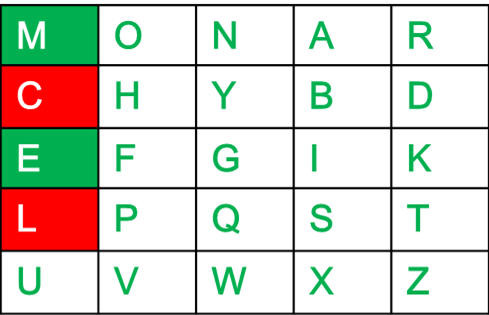•
• If both the letters are in the same row: Take the letter to the right of each one (going back to the leftmost if at the rightmost position).
For example:

Diagraph: "st"
Encrypted Text: tl
Encryption:
s -> t
t -> l
•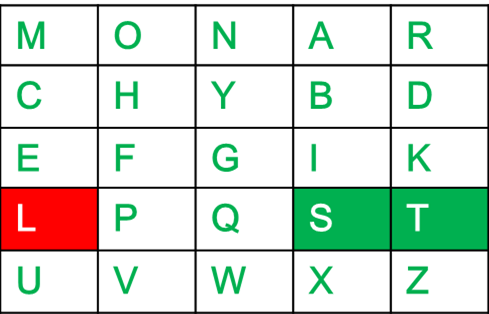•
• If neither of the above rules is true: Form a rectangle with the two letters and take the letters on the horizontal opposite corner of the rectangle.
For example:

Diagraph: "nt"
Encrypted Text: rq
Encryption:
n -> r
t -> q
•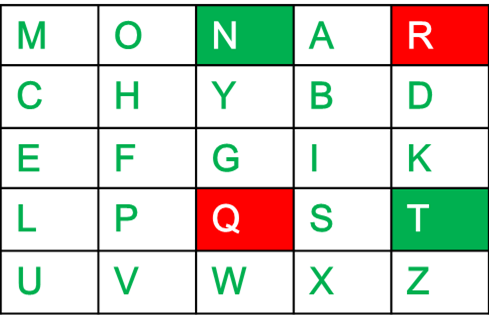•

For example:

Plain Text: "instrumentsz"
Encrypted Text: gatlmzclrqtx
Encryption:
i -> g
n -> a
s -> t
t -> l
r -> m
u -> z
m -> c
e -> l
n -> r
t -> q
s -> t
z -> x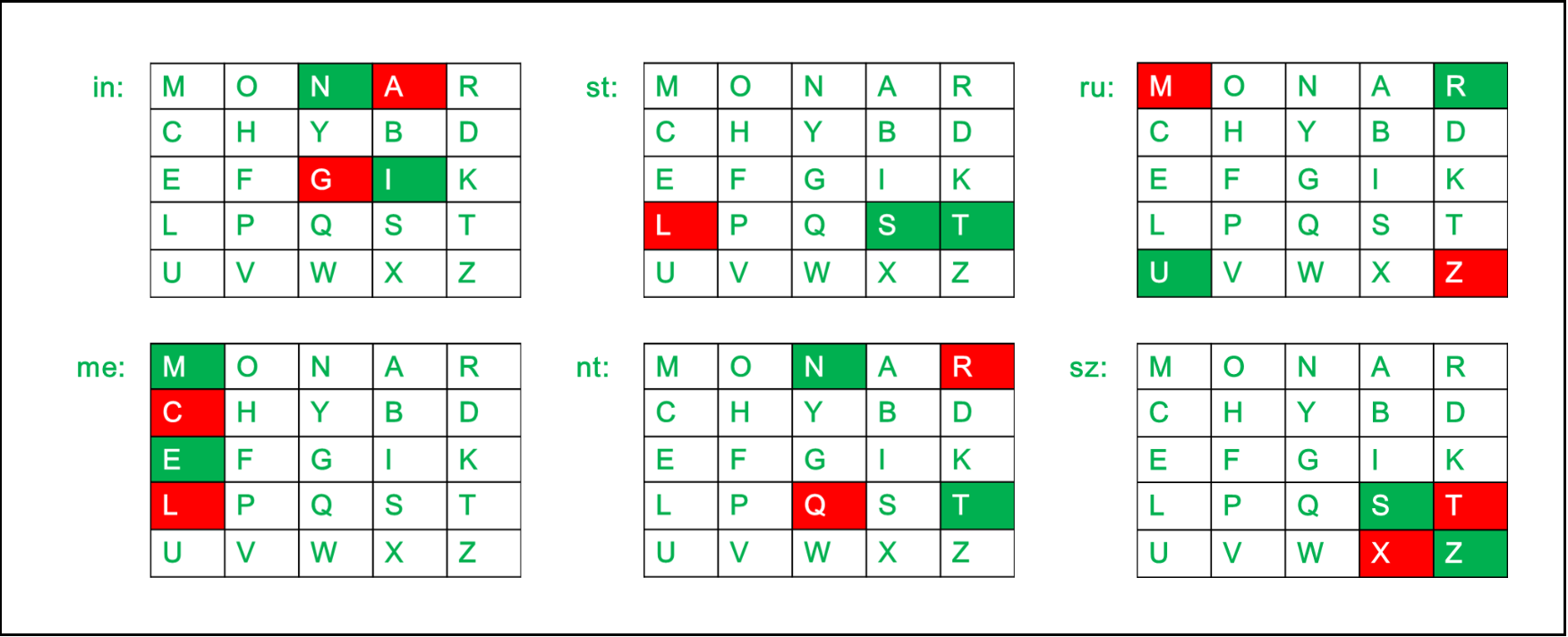Below is an implementation of Playfair Cipher in C:

C

 // C program to implement Playfair Cipher   #include #include #include   #define SIZE 30   // Function to convert the string to lowercase void toLowerCase(char plain[], int ps) {     int i;     for (i = 0; i < ps; i++) {         if (plain[i] > 64 && plain[i] < 91)             plain[i] += 32;     } }   // Function to remove all spaces in a string int removeSpaces(char* plain, int ps) {     int i, count = 0;     for (i = 0; i < ps; i++)         if (plain[i] != ' ')             plain[count++] = plain[i];     plain[count] = '\0';     return count; }   // Function to generate the 5x5 key square void generateKeyTable(char key[], int ks, char keyT) {     int i, j, k, flag = 0, *dicty;       // a 26 character hashmap     // to store count of the alphabet     dicty = (int*)calloc(26, sizeof(int));     for (i = 0; i < ks; i++) {         if (key[i] != 'j')             dicty[key[i] - 97] = 2;     }       dicty['j' - 97] = 1;       i = 0;     j = 0;       for (k = 0; k < ks; k++) {         if (dicty[key[k] - 97] == 2) {             dicty[key[k] - 97] -= 1;             keyT[i][j] = key[k];             j++;             if (j == 5) {                 i++;                 j = 0;             }         }     }       for (k = 0; k < 26; k++) {         if (dicty[k] == 0) {             keyT[i][j] = (char)(k + 97);             j++;             if (j == 5) {                 i++;                 j = 0;             }         }     } }   // Function to search for the characters of a digraph // in the key square and return their position void search(char keyT, char a, char b, int arr[]) {     int i, j;       if (a == 'j')         a = 'i';     else if (b == 'j')         b = 'i';       for (i = 0; i < 5; i++) {           for (j = 0; j < 5; j++) {               if (keyT[i][j] == a) {                 arr = i;                 arr = j;             }             else if (keyT[i][j] == b) {                 arr = i;                 arr = j;             }         }     } }   // Function to find the modulus with 5 int mod5(int a) { return (a % 5); }   // Function to make the plain text length to be even int prepare(char str[], int ptrs) {     if (ptrs % 2 != 0) {         str[ptrs++] = 'z';         str[ptrs] = '\0';     }     return ptrs; }   // Function for performing the encryption void encrypt(char str[], char keyT, int ps) {     int i, a;       for (i = 0; i < ps; i += 2) {           search(keyT, str[i], str[i + 1], a);           if (a == a) {             str[i] = keyT[a][mod5(a + 1)];             str[i + 1] = keyT[a][mod5(a + 1)];         }         else if (a == a) {             str[i] = keyT[mod5(a + 1)][a];             str[i + 1] = keyT[mod5(a + 1)][a];         }         else {             str[i] = keyT[a][a];             str[i + 1] = keyT[a][a];         }     } }   // Function to encrypt using Playfair Cipher void encryptByPlayfairCipher(char str[], char key[]) {     char ps, ks, keyT;       // Key     ks = strlen(key);     ks = removeSpaces(key, ks);     toLowerCase(key, ks);       // Plaintext     ps = strlen(str);     toLowerCase(str, ps);     ps = removeSpaces(str, ps);       ps = prepare(str, ps);       generateKeyTable(key, ks, keyT);       encrypt(str, keyT, ps); }   // Driver code int main() {     char str[SIZE], key[SIZE];       // Key to be encrypted     strcpy(key, "Monarchy");     printf("Key text: %s\n", key);       // Plaintext to be encrypted     strcpy(str, "instruments");     printf("Plain text: %s\n", str);       // encrypt using Playfair Cipher     encryptByPlayfairCipher(str, key);       printf("Cipher text: %s\n", str);       return 0; }   // This code is contributed by AbhayBhat

Output

Key text: Monarchy
Plain text: instruments
Cipher text: gatlmzclrqtx
Decryption Technique

Decrypting the Playfair cipher is as simple as doing the same process in reverse. The receiver has the same key and can create the same key table, and then decrypt any messages made using that key.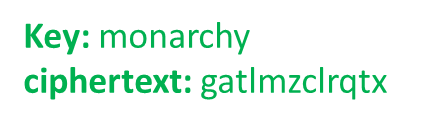The Playfair Cipher Decryption Algorithm:
The Algorithm consistes of 2 steps:

1. Generate the key Square(5×5) at the receiver’s end:
• The key square is a 5×5 grid of alphabets that acts as the key for encrypting the plaintext. Each of the 25 alphabets must be unique and one letter of the alphabet (usually J) is omitted from the table (as the table can hold only 25 alphabets). If the plaintext contains J, then it is replaced by I.

• The initial alphabets in the key square are the unique alphabets of the key in the order in which they appear followed by the remaining letters of the alphabet in order.

2. Algorithm to decrypt the ciphertext: The ciphertext is split into pairs of two letters (digraphs).

Note: The ciphertext always have even number of characters.

1. For example:

CipherText: "gatlmzclrqtx"
After Split: 'ga' 'tl' 'mz' 'cl' 'rq' 'tx'
1. Rules for Decryption:
• If both the letters are in the same column: Take the letter above each one (going back to the bottom if at the top).
For example:

Diagraph: "cl"
Decrypted Text: me
Decryption:
c -> m
l -> e
••
• If both the letters are in the same row: Take the letter to the left of each one (going back to the rightmost if at the leftmost position).
For example:

Diagraph: "tl"
Decrypted Text: st
Decryption:
t -> s
l -> t
••
• If neither of the above rules is true: Form a rectangle with the two letters and take the letters on the horizontal opposite corner of the rectangle.
For example:

Diagraph: "rq"
Decrypted Text: nt
Decryption:
r -> n
q -> t
••

For example:

Plain Text: "gatlmzclrqtx"
Decrypted Text: instrumentsz
Decryption:
(red)-> (green)
ga -> in
tl -> st
mz -> ru
cl -> me
rq -> nt
tx -> szBelow is an implementation of Playfair Cipher Decryption in C:

C

 #include #include #include #define SIZE 30   // Convert all the characters // of a string to lowercase void toLowerCase(char plain[], int ps) {     int i;     for (i = 0; i < ps; i++) {         if (plain[i] > 64 && plain[i] < 91)             plain[i] += 32;     } }   // Remove all spaces in a string // can be extended to remove punctuation int removeSpaces(char* plain, int ps) {     int i, count = 0;     for (i = 0; i < ps; i++)         if (plain[i] != ' ')             plain[count++] = plain[i];     plain[count] = '\0';     return count; }   // generates the 5x5 key square void generateKeyTable(char key[], int ks, char keyT) {     int i, j, k, flag = 0, *dicty;       // a 26 character hashmap     // to store count of the alphabet     dicty = (int*)calloc(26, sizeof(int));       for (i = 0; i < ks; i++) {         if (key[i] != 'j')             dicty[key[i] - 97] = 2;     }     dicty['j' - 97] = 1;       i = 0;     j = 0;     for (k = 0; k < ks; k++) {         if (dicty[key[k] - 97] == 2) {             dicty[key[k] - 97] -= 1;             keyT[i][j] = key[k];             j++;             if (j == 5) {                 i++;                 j = 0;             }         }     }     for (k = 0; k < 26; k++) {         if (dicty[k] == 0) {             keyT[i][j] = (char)(k + 97);             j++;             if (j == 5) {                 i++;                 j = 0;             }         }     } }   // Search for the characters of a digraph // in the key square and return their position void search(char keyT, char a, char b, int arr[]) {     int i, j;       if (a == 'j')         a = 'i';     else if (b == 'j')         b = 'i';       for (i = 0; i < 5; i++) {         for (j = 0; j < 5; j++) {             if (keyT[i][j] == a) {                 arr = i;                 arr = j;             }             else if (keyT[i][j] == b) {                 arr = i;                 arr = j;             }         }     } }   // Function to find the modulus with 5 int mod5(int a) {     if (a < 0)         a += 5;     return (a % 5); }   // Function to decrypt void decrypt(char str[], char keyT, int ps) {     int i, a;     for (i = 0; i < ps; i += 2) {         search(keyT, str[i], str[i + 1], a);         if (a == a) {             str[i] = keyT[a][mod5(a - 1)];             str[i + 1] = keyT[a][mod5(a - 1)];         }         else if (a == a) {             str[i] = keyT[mod5(a - 1)][a];             str[i + 1] = keyT[mod5(a - 1)][a];         }         else {             str[i] = keyT[a][a];             str[i + 1] = keyT[a][a];         }     } }   // Function to call decrypt void decryptByPlayfairCipher(char str[], char key[]) {     char ps, ks, keyT;       // Key     ks = strlen(key);     ks = removeSpaces(key, ks);     toLowerCase(key, ks);       // ciphertext     ps = strlen(str);     toLowerCase(str, ps);     ps = removeSpaces(str, ps);       generateKeyTable(key, ks, keyT);       decrypt(str, keyT, ps); }   // Driver code int main() {     char str[SIZE], key[SIZE];       // Key to be encrypted     strcpy(key, "Monarchy");     printf("Key text: %s\n", key);       // Ciphertext to be decrypted     strcpy(str, "gatlmzclrqtx");     printf("Plain text: %s\n", str);       // encrypt using Playfair Cipher     decryptByPlayfairCipher(str, key);       printf("Deciphered text: %s\n", str);       return 0; }   // This code is contributed by AbhayBhat

Output

Key text: Monarchy
Plain text: gatlmzclrqtx
Deciphered text: instrumentsz
1. It is significantly harder to break since the frequency analysis technique used to break simple substitution ciphers is difficult but still can be used on (25*25) = 625 digraphs rather than 25 monographs which is difficult.

2. Frequency analysis thus requires more cipher text to crack the encryption.